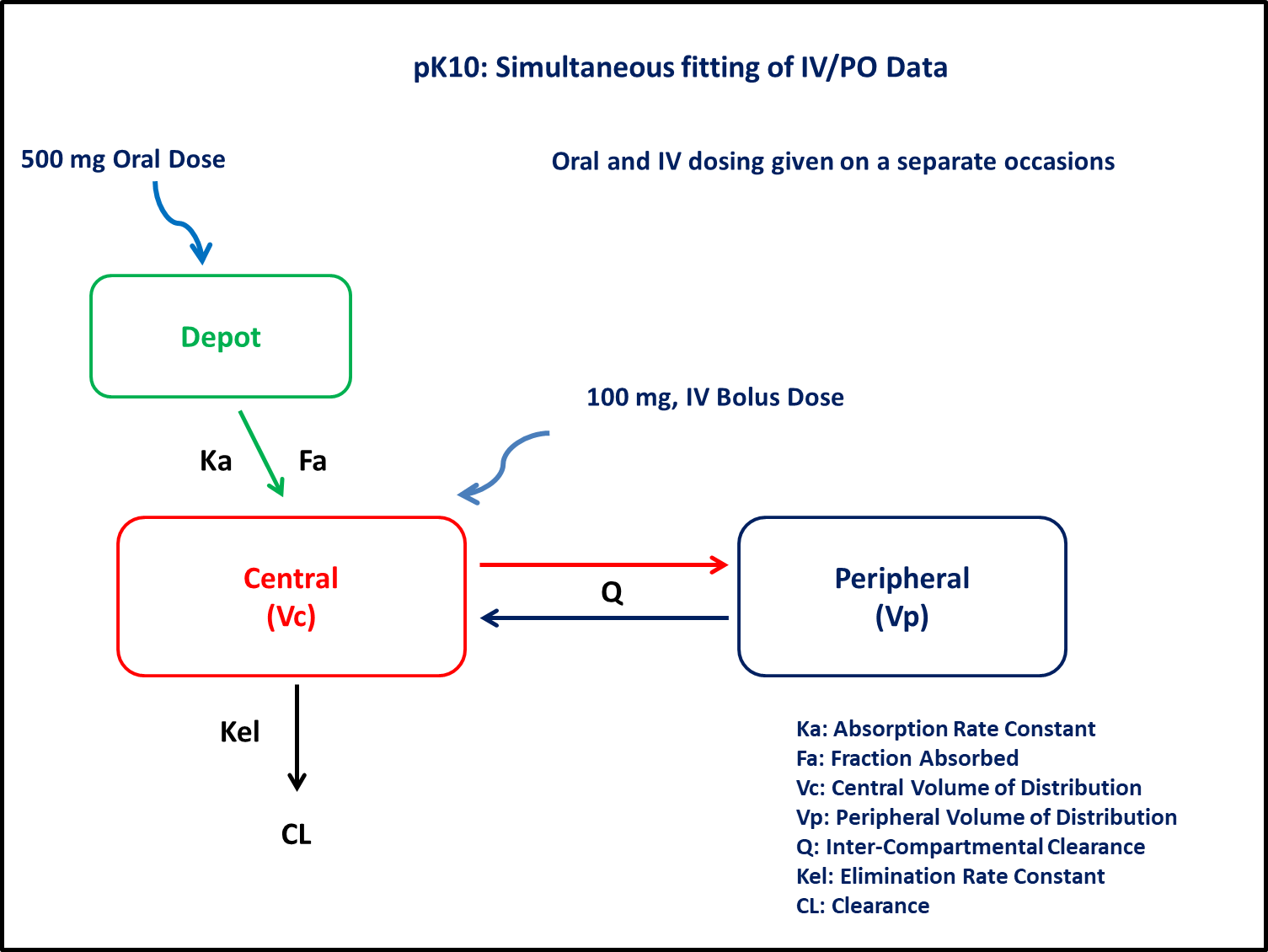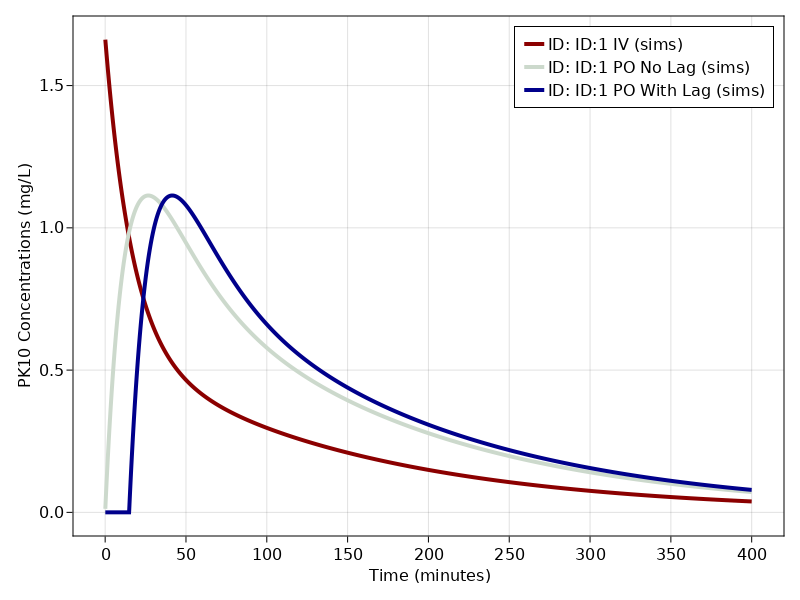# Exercise PK10 - Simultaneous fitting of IV/PO data

### Background

• Structural model - Two compartment linear elimination with first order absorption

• Route of administration - IV bolus and Oral given on separate occasions

• Dosage regimens - 100mg IV Bolus and 500mg Oral

• Subject - 1### Learning Outcome

In this model, Simultaneous fitting of iv/po data will help you to understand the disposition of drug following iv and oral administration (with / without lag time).

### Objectives

In this tutorial, you will learn how to build a two compartment model and to simulate the model for a single subject.

### Libraries

Call the "necessary" libraries to get start.

using Random
using Pumas
using PumasUtilities
using CairoMakie


### Model

In this two compartment model, we administer dose to Depot and Central compartment.

pk_10           = @model begin
desc        = "Two Compartment Model"
timeu       = u"minute"
end

@param begin
"Volume of Central Compartment (L)"
tvvc        ∈ RealDomain(lower=0)
"Volume of Peripheral Compartment (L)"
tvvp        ∈ RealDomain(lower=0)
"InterCompartmental Clearance (L/min)"
tvq         ∈ RealDomain(lower=0)
"Clearance (L/min)"
tvcl        ∈ RealDomain(lower=0)
"Absorption Rate Constant (min⁻¹)"
tvka        ∈ RealDomain(lower=0)
"Fraction of drug absorbed"
tvfa        ∈ RealDomain(lower=0)
"Lagtime (min)"
tvlag       ∈ RealDomain(lower=0)
Ω           ∈ PDiagDomain(7)
"Proportional RUV"
σ²_prop     ∈ RealDomain(lower=0)
end

@random begin
η           ~ MvNormal(Ω)
end

@pre begin
Vc          = tvvc * exp(η)
Vp          = tvvp * exp(η)
Q           = tvq * exp(η)
CL          = tvcl * exp(η)
Ka          = tvka * exp(η)
end

@dosecontrol begin
bioav       = (Depot=tvfa * exp(η),)
lags        = (Depot=tvlag * exp(η),)
end

@dynamics Depots1Central1Periph1

@derived begin
cp          = @. Central/Vc
"""
Observed Concentration (mg/L)
"""
dv          ~ @. Normal(cp, sqrt(cp^2*σ²_prop))
end
end

PumasModel
Parameters: tvvc, tvvp, tvq, tvcl, tvka, tvfa, tvlag, Ω, σ²_prop
Random effects: η
Covariates:
Dynamical variables: Depot, Central, Peripheral
Derived: cp, dv
Observed: cp, dv


### Parameters

Parameters provided for simulation. tv represents the typical value for parameters.

• $Vc$ - Volume of Central Compartment (L)

• $Vp$ - Volume of Peripheral Compartment (L)

• $Q$ - InterCompartmental clearance (L/min)

• $Cl$ - Clearance from Central InterCompartmental (L/min)

• $Ka$ - Absorption rate constant (min⁻¹)

• $Fa$ - Fraction of drug absorbed

• $lags$ - Lagtime (min)

• $Ω$ - Between Subject Variability

• $σ$ - Residual error

##### IV / PO - without lagtime
param1 = ( tvvc    = 59.9348,
tvvp    = 60.5898,
tvq     = 1.55421,
tvcl    = 0.967573,
tvka    = 0.0471557,
tvfa    = 0.318748,
tvlag   = 0,
Ω       = Diagonal([0.0,0.0,0.0,0.0,0.0,0.0,0.0,0.0]),
σ²_prop = 0.01)

(tvvc = 59.9348, tvvp = 60.5898, tvq = 1.55421, tvcl = 0.967573, tvka = 0.0
471557, tvfa = 0.318748, tvlag = 0, Ω = [0.0 0.0 … 0.0 0.0; 0.0 0.0 … 0.0 0
.0; … ; 0.0 0.0 … 0.0 0.0; 0.0 0.0 … 0.0 0.0], σ²_prop = 0.01)

##### IV / PO - with lagtime
param2 = (param1..., tvlag = 14.8187)

(tvvc = 59.9348, tvvp = 60.5898, tvq = 1.55421, tvcl = 0.967573, tvka = 0.0
471557, tvfa = 0.318748, tvlag = 14.8187, Ω = [0.0 0.0 … 0.0 0.0; 0.0 0.0 …
0.0 0.0; … ; 0.0 0.0 … 0.0 0.0; 0.0 0.0 … 0.0 0.0], σ²_prop = 0.01)


### DosageRegimen

DosageRegimen - Single Subject receiving 100mg Intravenous Bolus dose and 500mg Oral dose on different occasion.

##### IV
ev1     = DosageRegimen(100, time = 0, cmt = 2)
sub1_iv = Subject(id = "ID:1 IV", events = ev1, observations = (cp = nothing,))

Subject
ID: ID:1 IV
Events: 1
Observations: cp: (nothing)

##### PO
ev2     = DosageRegimen(500, time = 0, cmt = 1)
ids = ["ID:1 PO No Lag", "ID:1 PO With Lag"]
pop_po = map(i -> Subject(id = ids[i], events = ev2, observations = (cp = nothing,)), 1:length(ids))

Population
Subjects: 2
Observations: cp


### Simulation

##### IV

Lets simulate plasma concentration with specific observation times after IV bolus.

Random.seed!(123)
sim_iv_sub1 = simobs(pk_10, sub1_iv, param1, obstimes = 0.1:0.1:400)

##### PO

Lets simulate plasma concentration with specific observation times after PO (with/without lagtime)

Random.seed!(123)
sim_po_sub1 = map(zip(pop_po, [param1, param2])) do (subj, p)
return simobs(pk_10, subj, p, obstimes = 0.1:0.1:400)
end


### Visualization

all_sims = [sim_iv_sub1, sim_po_sub1...]

f, a, p = sim_plot(pk_10, all_sims,
observations = :cp,
color = :redsblues,
linewidth = 4,
axis = (xlabel = "Time (minutes)",
ylabel = "PK10 Concentrations (mg/L)",
xticks = 0:50:400))
axislegend(a)
f